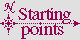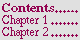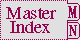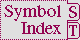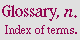#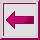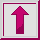Accessor VALUES

Syntax:

values &rest object => object*

(setf (values &rest place) new-values)

Arguments and Values:

object---an object.

place---a place.

new-value---an object.

Description:

values returns the objects as multiple values.

setf of values is used to store the multiple values new-values into the places. See Section 5.1.2.3 (VALUES Forms as Places).

Examples:

``` (values) =>  <no values>
(values 1) =>  1
(values 1 2) =>  1, 2
(values 1 2 3) =>  1, 2, 3
(values (values 1 2 3) 4 5) =>  1, 4, 5
(defun polar (x y)
(values (sqrt (+ (* x x) (* y y))) (atan y x))) =>  POLAR
(multiple-value-bind (r theta) (polar 3.0 4.0)
(vector r theta))
=>  #(5.0 0.927295)
```

Sometimes it is desirable to indicate explicitly that a function returns exactly one value. For example, the function

``` (defun foo (x y)
(floor (+ x y) y)) =>  FOO
```
returns two values because floor returns two values. It may be that the second value makes no sense, or that for efficiency reasons it is desired not to compute the second value. values is the standard idiom for indicating that only one value is to be returned:

``` (defun foo (x y)
(values (floor (+ x y) y))) =>  FOO
```
This works because values returns exactly one value for each of args; as for any function call, if any of args produces more than one value, all but the first are discarded.

Affected By: None.

Exceptional Situations: None.